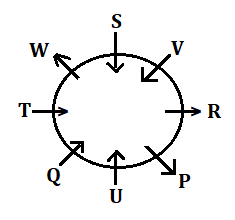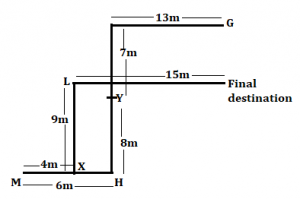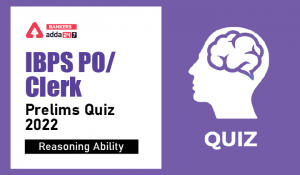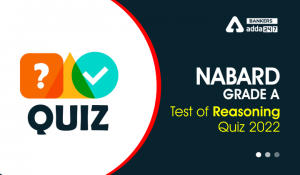Latest Banking jobs   »   Reasoning Ability Quiz for Prelims Exams-...

# Reasoning Ability Quiz for Prelims Exams- SBI & IBPS 2020- 2nd December

Directions (1-5): Study the information carefully and answer the questions given below.
Eight people P, Q, R, S, T, U, V and W are sitting in a circular table. Some of them facing towards center and some of them facing outside the center but not necessarily in the same order.
R sits second to the right of U. One person sits between R and S. T sits third to the right of V. T face opposite direction of R. T is not immediate neighbor of S and U. V sits second to the left of P, who sits second to the right of Q. Q and P are not immediate neighbors of S. W and S sit immediate right to each other. R faces the same direction as P. T faces towards the center.

Q1.Who among the following sits second to the right of V?
(a) P
(b) W
(c) T
(d) S
(e) None of these

Q2. Who among the following sits opposite to T?
(a) P
(b) U
(c) W
(d) T
(e) R

Q3.Who among the following sits immediate right of Q?
(a) U
(b) T
(c) P
(d) W
(e) None of these

Q4. Four of the following five are alike in a certain way based and from a group, find the one that does not belong to that group?
(a) Q
(b) U
(c) T
(d) P
(e) S

Q5. How many persons sit between P and S when counted to the right of P?
(a) Two
(b) Three
(c) Four
(d) Five
(e) None

Direction (6-10): In these questions, relationship between different elements is show in the statements. The statements are followed by conclusions. Study the conclusions based on the given statements and select the appropriate answer:
(a) If only conclusion I follows.
(b) If only conclusion II follows.
(c) If either conclusion I or II follows
(d) If neither conclusion I nor II follows.
(e) If both conclusions I and II follow.

Q6. Statements: W=E>F>V, M≥W, G≥S≥V
Conclusion: I: E≤M             II: M>S

Q7. Statements: H≤R, T<U≤H, H<I<R
Conclusion: I: I≥T              II: I>T

Q8. Statements: R>S, P>M≥O, R=Q<O
Conclusion: I: M> R            II: S<O

Q9. Statements: B<E<R<T, U≥Y=T, U=X
Conclusion: I: B>Y             II: X>E

Q10. Statements: S<M<U, Y>X>R, T≥R, Y<U
Conclusion: I: X>M             II: M≥X

Directions (11-15): Study the information carefully and answer the questions given below.
A person start walking in west direction from point G after walking 13m he takes a left turn and walks 7m to reach point Y. From point Y, he walks 8m in south direction to reach point H. From point H, He takes right turn and walks 6m to reach point M. Now he starts walking in east direction and walks 4m to reach at point X. From point X, He takes left turn and walks 9m to reach point L. Finally he takes a right turn and walks 15m to reach its final destination.

Q11. What is the shortest distance between final point and G?
(a) 7m
(b) 8m
(c) 5m
(d) 4m
(e) None of these

Q12. What is the shortest distance between Point X and point Y?
(a) 2√34 m
(b) 68m
(c) 2√17m
(d)√67m
(e) None of these

Q13. In which direction point X with respect to point G?
(a) Northeast
(b) East
(c) Southwest
(d) South
(e) None of these

Q14. In which direction point Y with respect to final point?
(a) West
(b) East
(c) South
(d) Northeast
(e) None of these

Q15. If point R is 6m east of point Y then what is the shortest distance between point R and point H?
(a) 10m
(b) 8m
(c) 12m
(d) 11m
(e) None of these

Practice More Questions of Reasoning for Competitive Exams:

Reasoning for Competitive Exams
###### Reasoning Ability Quiz for IBPS 2020 Mains Exams- 27th November
Prelims Quiz Study Plan for SBI & IBPS Exams 2020

Solutions

Solution (1-5):
Sol.S1.Ans(b)
S2.Ans(e)
S3.Ans(a)
S4.Ans(d)
S5.Ans(c)

Solutions (6-10):
S6. Ans(a)
Sol. I: E≤M(true)   II: M>S(False)
S7. Ans(b)
Sol. I: I≥T (False)   II: I>T (True)
S8. Ans(e)
Sol. I: M>R (True)  II: S<O (True)
S9. Ans(b)
Sol. I: B>Y (False)  II: X>E (True)
S10. Ans(c)
Sol. I: X>M (False)  II: M≥X (False)

Solution (11-15):

Sol.S11.Ans(e)
S12.Ans(c)
S13.Ans(c)
S14.Ans(e)
S15.Ans(a)

#### Download PDF of this Reasoning Quiz for IBPS & SBI Prelims 2020

Practice with Crash Course and Online Test Series for IBPS Clerk Prelims:

#### If you are preparing for IBPS & SBI Prelims Exam, then you can also check out a video for Reasoning below:#### Congratulations!Incorrect details? Fill the form again here

•Reasoning Ability Quiz For IBPS Clerk/PO...
•Reasoning Ability Quiz For IBPS Clerk/PO...
•Reasoning Ability Quiz For IBPS RRB PO C...
•Reasoning Ability Quiz For IBPS RRB PO C...
•Reasoning Ability Quiz For NABARD Grade ...
•Reasoning Ability Quiz For IBPS RRB PO C...